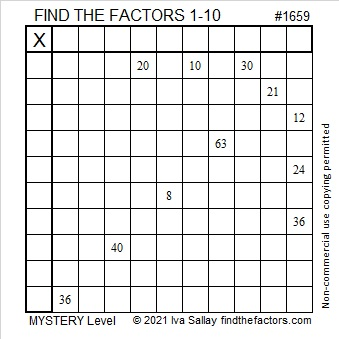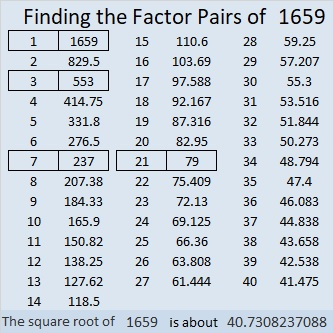# 1659 Another Mystery

Contents

### Today’s Puzzle:

Both 20, 10, 30 and 12, 24, 36 have two possible common factors that will only put numbers from 1 to 10 in the first column and the top row of this mystery level puzzle. However, the puzzle has only one solution. Examine all the clues in the puzzle and think logically to determine what those common factors must be.### Factors of 1659:

• 1659 is a composite number.
• Prime factorization: 1659 = 3 × 7 × 79.
• 1659 has no exponents greater than 1 in its prime factorization, so √1659 cannot be simplified.
• The exponents in the prime factorization are 1, 1, and 1. Adding one to each exponent and multiplying we get (1 + 1)(1 + 1)(1 + 1) = 2 × 2 × 2 = 8. Therefore 1659 has exactly 8 factors.
• The factors of 1659 are outlined with their factor pair partners in the graphic below.### More About the Number 1659:

1659 is the difference of two squares in FOUR different ways:
830² – 829² = 1659,
278² – 275² = 1659,
122² – 115² = 1659, and
50² – 29² = 1659.

This site uses Akismet to reduce spam. Learn how your comment data is processed.